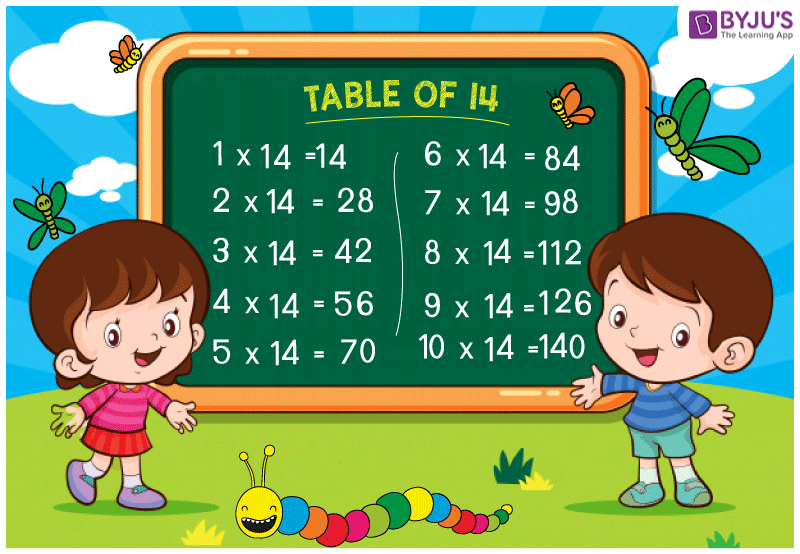# Table of 14

Table of 14 is provided here. The multiplication table is a mathematical table which is used to explain the multiplication operation. The multiplication table is very useful for the students (from class 6 to 12) as well as for the applicants, preparing for UPSC, SSC, and other competitive exams.

It is advised to memorise the multiplication table of 14 so that students can solve multiplication problems easily. Memorising the 14 times table saves lots of time when you have to solve more number of question in a very short interval of time.Here we have provided the table of 14 so that students can go through it whenever they are facing any difficulties.

## Table of 14 Charts

 14 × 1 = 14 14 × 2 = 28 14 × 3 = 42 14 × 4 = 56 14 × 5 = 70 14 × 6 = 84 14 × 7 = 98 14 × 8 = 112 14 × 9 = 126 14 × 10 = 140 14 × 11 = 154 14 × 12 = 168 14 × 13 = 182 14 × 14 = 196 14 × 15 = 210 14 × 16 = 224 14 × 17 = 238 14 × 18 = 252 14 × 19 = 266 14 × 20 = 280

Visit BYJU’S for multiplication tables, the latest CBSE study materials. We also provide subject wise interacting and engaging videos.

Get More Maths Tables:-

#### 1 Comment

1. Rishita Shukla

Thank you! I had a test coming up and I forgot to learn for it , this really helped!!!!!!!!!!!!!!